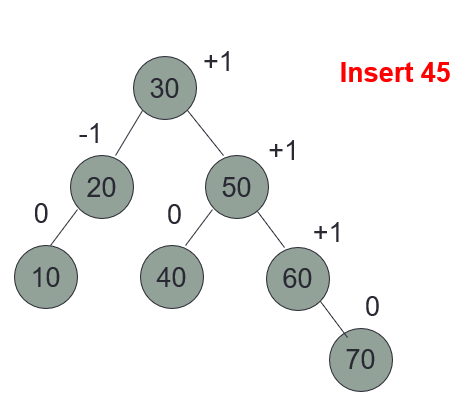top of page
Search

# What Is AVL Tree | Get Help In Data Structure AlgorithmsGo through below points to know clear explanation about AVL Tree:

• Height: the longest path from a node to a leaf node.

• Height-balanced tree: A binary tree is a height-balanced-p-tree if for each node in the tree, the absolute difference in height of its two subtrees is at most p.

AVL tree is a BST that is height-balanced-1-tree.

• For each node in the tree, the absolute difference in height of its two subtrees must be at most 1.

• Balance = Right Subtree Height Left Subtree Height

• Therefore, it must be either +1 (longer right), 0 (equal), -1 (longer left).### AVL Tree Specification

Elements: The elements are nodes, each node contains the following data type: Type.

Structure: Same as for the BST; in addition the height difference of the two subtrees of any node is at the most one.

Domain: the number of nodes in a AVL is bounded; type AVLTree.

Operations:

1. Method FindKey (int tkey, boolean found).

2. Method Insert (int k, Type e, boolean inserted).

3. Method Remove_Key (int tkey, boolean deleted)

4. Method Update(Type e)

5. Method Traverse (Order ord)

6. Method DeleteSub ( )

7. Method Retrieve (Type e)

8. Method Empty (boolean empty).

9. Method Full (boolean full)

### AVL Tree Element

```public class AVLNode<T> {
public int key
public T data;
public Balance bal;	// Balance is enum (+1, 0, -1)
public AVLNode<T> left, right;

public AVLNode(int key, T data) {
this.key = key;
this.data = data;
bal = Balance.Zero;
left = right = null;
}
...
...
}

```

### AVL Tree Implementation

• The implementation of: FindKey, Update data, Traverse, Retrieve, Empty, Full, and any other method that doesn’t change the tree are exactly like the implementation of BST.

• The only difference in implementation is when we change the nodes of the tree, i.e. Insert/Remove from the tree.

### AVL Tree Insert

Step 1: A node is first inserted into the tree as in a BST.

Step 2: Nodes in the search path are examined to see if there is a pivot node. Three cases arise.

• search path is a unique path from the root to the new node.

• pivot node is a node closest to the new node on the search path, whose balance is either –1 or +1.

Case 1: There is no pivot node in the search path. No adjustment required.

Case 2: The pivot node exists and the subtree to which the new node is added has smaller height. No adjustment required.

Case 3: The pivot node exists and the subtree to which the new node is added has the larger height. Adjustment required.

Case 1: Insert 40Case 1: Insert 55Case 2: Insert 5Case 2: Insert 45Case 2: Insert 45Insert Case 3:

• When after an insertion or a deletion an AVL tree becomes imbalanced, adjustments must be made to the tree to change it back into an AVL tree.

• These adjustments are called rotations.

• Rotations can be in the left or right direction.

• Rotations are either single or double rotations

Therefore, there are four different rotations:

• Left Rotation (Single)

• Right Rotation (Single)

• Left-Right Rotations (Double)

• Right-Left Rotations (Double)

### AVL Tree Delete

Step 1: Delete the node as in BSTs. Remember there are three cases for BST deletion.

Step 2: For each node on the path from the root to deleted node, check if the node has become imbalanced; if yes perform rotation operations otherwise update balance factors and exit. Three cases can arise for each node p, in the path.

Case 1: Node p has balance factor 0. No adjustment required.

Case 2: Node p has balance factor of +1 or –1 and a node was deleted from the taller sub-trees. No adjustment required.

Case 3: Node p has balance factor of +1 or –1 and a node was deleted from the shorter sub-trees. Adjustment required.

Case 1: Delete 60Case 1: Delete 50